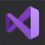# What is the value of $\zeta (4)$?

We know $\zeta (n) = \frac{1}{1^n}+\frac{1}{2^n}+\frac{1}{3^n} + \cdots$ so $\zeta (4) = \frac{1}{1^4}+\frac{1}{2^4}+\frac{1}{3^4} + \cdots$ At last note I said $\frac{x}{1!}-\frac{x^3}{3!}+\frac{x^5}{5!}-\cdots=x(1-\frac{x^2}{\pi ^2})(1-\frac{x^2}{(2\pi)^2})(1-\frac{x^2}{(3\pi)^2})\cdots$ Therefore, the split items with $x^5$ items on the left and right are equal. In the left of the formula, it has $x^5 \frac{1}{5!}$ and in the right of the formula -- how many $x^5$ does it have? We must have $x^5$, remove the first $x$, it takes two $\frac{x^2}{\cdots}$ to form $x^5$. So we have: $\frac{1}{5!}=\frac{1}{\pi^2}(\frac{1}{(2\pi)^2}+\frac{1}{(3\pi)^2}+\cdots)+\frac{1}{(2\pi)^2}(\frac{1}{(3\pi)^2}+\frac{1}{(4\pi)^2}+\cdots)+\cdots$ $\frac{\pi^4}{5!}=\frac{1}{1^2}(\frac{1}{2^2}+\frac{1}{3^2}+\cdots)+\frac{1}{2^2}(\frac{1}{3^2}+\frac{1}{4^2}+\cdots)+\cdots$ Let $S_1=\frac{1}{1^2}+\frac{1}{2^2}+\frac{1}{3^2}+\cdots = \frac{\pi^2}{6}$ then $\frac{\pi^4}{120}=\frac{1}{1^2}(S_1-\frac{1}{1^2})+\frac{1}{2^2}(S_1-\frac{1}{1^2}-\frac{1}{2^2})+\cdots$ $\frac{\pi^4}{120}=S_1(\frac{1}{1^2}+\frac{1}{2^2}+\cdots)-(\frac{1}{1^4}+\frac{1}{2^4}+\cdots)-\frac{1}{1^2}(\frac{1}{2^2}+\frac{1}{3^2}+\cdots)-\frac{1}{2^2}(\frac{1}{3^2}+\frac{1}{4^2}+\cdots)-\cdots$ $\frac{\pi^4}{120}=S_1^2-(\frac{1}{1^4}+\frac{1}{2^4}+\cdots)-[\frac{1}{1^2}(S_1-\frac{1}{1^2})+\frac{1}{2^2}(S_1-\frac{1}{1^2}-\frac{1}{2^2})+\cdots]$ $\frac{\pi^4}{120}=\frac{\pi^4}{36}-\zeta(4)-\frac{\pi^4}{120}$ $\zeta(4)=\frac{\pi^4}{36} - (\frac{\pi^4}{120} + \frac{\pi^4}{120})$ $\zeta(4)=\frac{\pi^4}{36}-\frac{\pi^4}{60}$ $\zeta(4)=\frac{\pi^4}{90}$Note by Raymond Fang
6 months ago

This discussion board is a place to discuss our Daily Challenges and the math and science related to those challenges. Explanations are more than just a solution — they should explain the steps and thinking strategies that you used to obtain the solution. Comments should further the discussion of math and science.

When posting on Brilliant:

• Use the emojis to react to an explanation, whether you're congratulating a job well done , or just really confused .
• Ask specific questions about the challenge or the steps in somebody's explanation. Well-posed questions can add a lot to the discussion, but posting "I don't understand!" doesn't help anyone.
• Try to contribute something new to the discussion, whether it is an extension, generalization or other idea related to the challenge.

MarkdownAppears as
*italics* or _italics_ italics
**bold** or __bold__ bold
- bulleted- list
• bulleted
• list
1. numbered2. list
1. numbered
2. list
Note: you must add a full line of space before and after lists for them to show up correctly
paragraph 1paragraph 2

paragraph 1

paragraph 2

[example link](https://brilliant.org)example link
> This is a quote
This is a quote
    # I indented these lines
# 4 spaces, and now they show
# up as a code block.

print "hello world"
# I indented these lines
# 4 spaces, and now they show
# up as a code block.

print "hello world"
MathAppears as
Remember to wrap math in $$ ... $$ or $ ... $ to ensure proper formatting.
2 \times 3 $2 \times 3$
2^{34} $2^{34}$
a_{i-1} $a_{i-1}$
\frac{2}{3} $\frac{2}{3}$
\sqrt{2} $\sqrt{2}$
\sum_{i=1}^3 $\sum_{i=1}^3$
\sin \theta $\sin \theta$
\boxed{123} $\boxed{123}$

Sort by:

Really, really interesting! Do more - keep inviting me as well!

- 6 months ago

:-)

- 6 months ago

Great ! For more higher terms use $\zeta(2n)= \dfrac{(-1)^{n+1} \beta_{2n}(2π)^{2n}}{2(2n)!}$ Or use your great way

- 6 months ago

:-)

- 6 months ago

What is the value of $\zeta(3)$? (This is an unsolved problem so far.)

- 6 months ago

One thing for sure - it's irrational!

- 6 months ago

$\zeta(3) = 1 + \frac{1}{2^3} + \frac{1}{3^3} + ... \approx 1.20205690315959428540$

The value of $\zeta(3)$ is called Apéry's constant

- 6 months ago

Thanks!

- 6 months ago

Generally $\sum_{n=1}^∞ f(n)= \int_1^∞ f(x)dx +\dfrac{f(z)+f(1)}{2} +\sum_{k=1}^∞ \dfrac{\beta_{2k}}{(2k)!}(\lim_{z\rightarrow{∞}} (f^{2k+1}(z) -f^{2k+1}(1)))$

Using this we can find the approximation

- 6 months ago